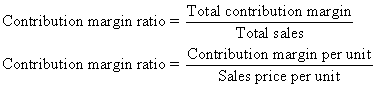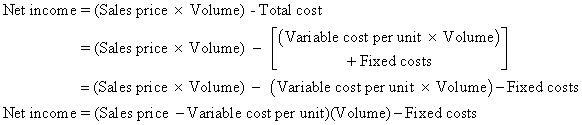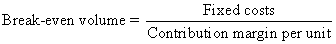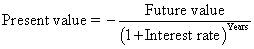## There are more accounting formulas than there is sand in the sea.

But every accounting formula is not created equal. Some accounting formulas are more important and fundamental than others.

As a small business, you have to keep abreast of all the transactions that are happening in your business. Sometimes this type of data can get overwhelming. You need a method to decipher what’s important and what’s not in your data

## Balance sheet formula

Assets – liabilities = equity (or assets = liabilities + equity)

This basic formula must stay in balance to generate an accurate balance sheet. This means that all accounting transactions must keep the formula in balance. If not, the accountant has made an error.

## Retained earnings formula

Beginning balance + net income – net losses – dividends = ending balance

## Income statement formula

Revenue (sales) – expenses = profit (or net income)

Keep in mind that revenue and sales may be used interchangeably. Profit and net income may also be used interchangeably. The income statement is also referred to as a profit and loss statement.

## Gross margin

Sales – cost of sales

Gross margin is not a company’s net income or profit. Other expenses, such as selling, general, and administrative (SG and A) expenses, are subtracted to arrive at net income.

## Operating income (earnings)

Gross profit – selling, general, and administrative (SG and A) expenses

## Statement of cash flows formula

Beginning cash balance + cash flow sources (uses) from operations + cash flow sources (uses) from financing + cash flow sources (uses) from investing = ending cash balance

This formula adds cash sources and subtracts cash uses.

## Inventory formula

Beginning inventory + purchases – cost of sales = ending inventory (or beginning inventory + purchases – ending inventory = cost of sales)

## Net sales formula

Gross sales – sales discounts – sales returns and allowances

## Book value of fixed (depreciable) assets

Original cost – accumulated depreciation

## Straight line depreciation

(Original cost – salvage value) / number of years in useful life

Salvage value is the dollar amount that the owner can receive for selling the asset at the end of its useful life.

## The accounting equation

The accounting equation equates assets with liabilities and owners’ equity:

Assets = Liability + Owners’ Equity

Assets are things owned by the company — such as cash, inventory, and equipment — that will provide some future benefit. Liabilities entail future sacrifices that the company must make, such as paying bills or other kinds of debts. Owners’ equity represents the portion of the company that actually belongs to the owner.

A basic rule of accounting is that the accounting equation must always balance. If assets exceed the sum of liabilities and owners’ equity, then the company holds things that don’t belong to anyone. If the sum of liabilities and owners’ equity exceeds assets, then owners and creditors lay claim to things that don’t exist.

## Net income

Net income is called the bottom line because in many ways it’s the sum total of accountants’ work. To calculate net income, subtract expenses from revenues:

Revenues – Expenses = Net income

Revenues are inflows and other kinds of sales to customers. Expenses are costs associated with making sales. Accountants also sometimes need to add gains or subtract losses in net income; these gains and losses come from miscellaneous events that affect stockholder value, such as selling equipment at a gain or getting your factory destroyed by a mutated prehistoric survivor of the dinosaurs.

## Cost of goods sold

For manufacturers and retailers, cost of goods sold measures how much the company paid — or will need to pay — for inventory items sold.

To compute a retailer’s cost of goods sold, use the following formula:

Beginning + Inputs = Outputs

Cost of beginning inventory + Cost of purchases – Cost of ending inventory = Costs of goods sold

Here, a retailer’s inputs are the cost of the purchases it makes. The outputs are the goods that were sold (recorded at cost, of course).

## Contribution margin

Contribution margin measures how selling one item, or a group of items, increases net income. To calculate contribution margin, subtract variable costs from sales:

Total sales – Total variable cost = Total contribution margin

Contribution margin helps managers by explaining how decisions will impact income. Should you prepare a special order with a contribution margin of \$100,000? Yes, because it will increase net income by \$100,000. Should you prepare another special order with a contribution margin of negative \$50,000? No, because it will decrease net income.

To compute contribution margin per unit, divide the total contribution margin by the number of units sold. Alternatively, you can calculate sales price less variable cost per unit:

Sales price – Variable cost per unit = Contribution margin per unit

To compute contribution margin ratio, divide contribution margin by sales, either in total or per unit:## Cost-volume profit analysis

Cost-volume-profit (CVP) analysis helps you understand how changes in volume affect costs and net income. If you know sales price, variable cost per unit, volume, and fixed costs, this formula will predict your net income:

Net income = (Sales price – Variable cost per unit)(Volume) – Fixed costs

First, understand where this formula comes from. Consider how production volume affects total costs:

Total cost = (Variable cost per unit x Volume) + Fixed costs

Variable cost per unit is the additional cost of producing a single unit. Volume is the number of units produced. Fixed cost is the total fixed cost for the period. Net income is just the difference between total sales and total cost:

Net income = (Sales price x Volume) – Total cost

Combining these two equations gives you the super-useful formula for understanding how volume affects profits:Not coincidentally, a critical part of this formula equals contribution margin — remember that sales price less variable cost per unit equals contribution margin per unit:

Sales price – Variable cost per unit = Contribution margin per unit

This formula lets you further simplify the CVP formula:

Net income = (Contribution margin x Volume) – Fixed costs

## Break-even analysis

Break-even analysis helps you determine how much you need to sell in order to break even — that is, to earn no net loss or profit. To figure out the break-even point, use this formula:Perhaps you recognize contribution margin in the denominator (Sales price – Variable cost per unit), allowing you to further simplify this formula:To figure out the number of units needed to break even, just divide total fixed costs by contribution margin per unit.

## Price variance

Price variance tells you how an unexpected change in the cost of direct materials affects total cost. Use this formula to compute price variance:

Price variance = (Standard price – Actual price) x Actual quantity

Standard price is the amount you originally expected to pay, per unit, of direct materials. Actual price is the real price you paid, per unit, for direct materials. The actual quantity is the number of units purchased and used in production.

Although the price variance formula focuses on the direct materials variance, you can easily adapt it to figure out the direct labor variance. To do so, replace standard price with the standard cost (per hour) of direct labor. Replace actual price with the actual cost (per hour) of direct labor. Then replace the actual quantity with the actual number of hours worked.

## Quantity variance

The direct materials quantity variance measures how using too much or too little in direct materials affects total costs. Stinginess in using direct materials should decrease your costs. However, wasting direct materials should increase costs. Here’s the formula:

Quantity variance = Standard price x (Standard quantity – Actual quantity)

Remember that standard price is how much you originally expected to pay, per unit, of direct materials. Standard quantity is the number of units of direct materials that you expected to use. Actual quantity is the number of units of direct materials that you actually used in production.

## Future value

Future value measures how much a present cash flow will be worth in the future. For example, if you put \$1,000 into the bank today, earning 6-percent interest a year, how much will you have ten years from now?

To solve these problems, many students use tables printed in textbooks or financial calculators. You can also solve these problems using the time value of money formula:

Future value = –Present value x (1 + interest rate)Years

Present value measures how much money you receive or pay now. Make this figure positive if you’re receiving the money and negative if you’re paying the money out. Future value is how much you can expect to receive or pay in the future (again, positive for incoming cash, negative for outgoing cash).

The interest rate should be put in as the annual interest rate (rather than daily, monthly, or quarterly). The number of years is for the period of time between the date of the present value and the date of the future value, in years.

Therefore, if present value equals –\$1,000, the interest rate is 6 percent, and the number of years is ten years.

Future value = –(–\$1,000) x (1 + 0.06)10

= \$1,000 x (1.06) 10

= \$1,000 x 1.791

= \$1.791

The future value indicates that, if you put \$1,000 away now, earning 6 percent, you can expect to receive \$1,791 at the end of ten years.

## Present value

Present value uses the same formula as future value.

Future value = –Present value x (1 + interest rate)Years

Here’s an example of how you can use this formula to compute the present value of a cash flow. Suppose that, four years from now, you want to have \$5,000 (that’s the future value). How much should you put into the bank today, earning 5-percent interest?So if you put \$4,114 into the bank today, earning 5-percent interest, then in four years you should have \$5,000 to take out.

Here’s a version of the formula to more directly compute present value:## 1. Overall value

The equation:

Total Assets = Liabilities + Equity

What this accounting equation includes:

• Assets are all of the things your company owns, including property, cash, inventory, accounts receivable, and any equipment that will allow you to produce a future benefit.
• Liabilities are obligations that it must pay, including things like lease payments, merchant account fees, accounts payable, and any other debt service.
• Equity is the portion of the company that actually belongs to the owner. If shareholders own the company, then stockholders’ equity would fall into this category as well.

This is a balance sheet equation. The dollar amount of assets on the left side of the equation must equal the sum of liabilities and equity on the right side of the equation.

## 2. Net income

The equation:

Net Income = Revenues – Expenses

What this accounting equation includes:

• Revenues are the sales or other positive cash inflow that comes into your company.
• Expenses are the costs incurred to generate revenue.

By subtracting your revenue from your expenses, you can calculate your net income. This is the money that you have earned at the end of the day. It’s possible that this number will demonstrate a net loss when your business is in its early stages. The ultimate goal of any business should be positive net income, which means your business is profitable.

## 3. Break-even point

The equation:

Break-Even Point = (Sales – Fixed Costs – Variable Costs = \$0 Profit)

What this accounting equation includes:

• Fixed Costs are recurring, predictable costs that you must pay to conduct business. These costs can include insurance premiums, rent, employee salaries, etc.
• Sales is the sales prices charged multiplied by the number of units sold.
• Variable Costs are any costs you incur that change based on the number of units produced or sold.

The break-even point tells you how much you need to sell to cover all of your costs and generate a profit of \$0. Every sale over the break-even point will generate a profit.

## 4. Cash ratio

The equation:

Cash Ratio = Cash ÷ Current Liabilities

What this accounting equation includes:

• Cash is the amount of cash you have at your disposal. This can include actual cash and cash equivalents, such as highly-liquid investment securities.
• Current Liabilities are the current debts the business has incurred.

This ratio gives you an idea of how much cash you currently have on hand. It also demonstrates how well your business can pay off its current liabilities. The higher the number, the healthier your company.

## 5. Profit margin

The equation:

Profit Margin = Net Income ÷ Sales

What this accounting equation includes:

• Sales refers to the operating revenue you generate from business activities.

When you divide your net income by your sales, you’ll get your organization’s profit margin. Your profit margin reports the net income earned on each dollar of sales. A high profit margin indicates a very healthy company. A low profit margin could indicate that your business does not handle expenses well.

Remember that your net income is made up of your total revenue minus your expenses. If you have high sales revenue but still have a low profit margin, it might be time to take a look at the figures making up your net income.

## 6. Debt-to-equity ratio

The equation:

Debt-to-Equity Ratio = Total Liabilities ÷ Total Equity

What this accounting equation includes:

• Total Liabilities include all of the costs you must pay to outside parties, such as accounts payable balances and interest, and principal payments on debt.
• Total Equity is how much of the company actually belongs to the owners. In other words, it’s the amount of money the owner has invested in his or her own company.

A high debt-to-equity ratio illustrates that a high proportion of your company’s financing comes from issuing debt, rather than issuing stock to shareholders. If you’re attempting to secure more financing or looking for investors, a high debt-to-equity ratio might make it more difficult to find creditors or investors who are willing to provide funds for your company.

## 7. Cost of goods sold

The equation:

Cost of Goods Sold = Beginning Inventory + Cost of Purchasing New Inventory – Ending Inventory

What this accounting equation includes:

• Beginning Inventory is how much inventory you have on hand at the beginning of the period.
• Cost of Purchasing new Inventory is the amount of money your company has to spend to secure the necessary products or materials to manufacture your products.
• Ending Inventory is the product you have remaining at the end of the period.

The costs of goods sold equation allows you to determine how much you spent to manufacturer the goods you sold. By subtracting the costs of goods sold from revenues, you’ll determine your gross profit.

## 8. Retained earnings equation

The equation:

Retained Earnings = Beginning Retained Earnings + Net Income or Net Loss – Cash Dividends

What the retained earnings formula includes:

• Retained Earnings represent the sum of all net income since business inception minus all cash dividends paid since inception.
• Beginning Retained Earnings are the retained earnings balance from the prior accounting period.
• The Company’s Net Income represents the balance after subtracting expenses from revenues. It’s also possible for this to result in a net loss.
• Cash Dividends are cash payouts to those who own common stock.

Knowing how to calculate retained earnings allows owners to perform a more in-depth financial analysis. The statement of retained earnings allows owners to analyze net income after accounting for dividend payouts. Owners should calculate the statement of retained earnings at the end of each accounting period, even if the amount of dividends issues was zero.

## Monitor your company’s financial health

A thorough accounting system and a well-maintained general ledger allow you to properly assess the financial health of your company. There are many more formulas that you can use, but the eight that we provided are some of the most important.

Although these equations seem straightforward, they can become more complicated in reality. Many small business owners find it much more challenging to balance the right side of the equation with the left side of the equation when factoring in the potentially hundreds of accounts they have in their company.